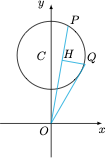# 每日一题构造图形

A．最大值为 $2$，最小值为 $\sqrt 3$

B．最大值为 $2$，最小值为 $1$

C．最大值为 $2\sqrt 3$，最小值为 $\sqrt 3$

D．最大值为 $2\sqrt 3$，最小值为 $1$